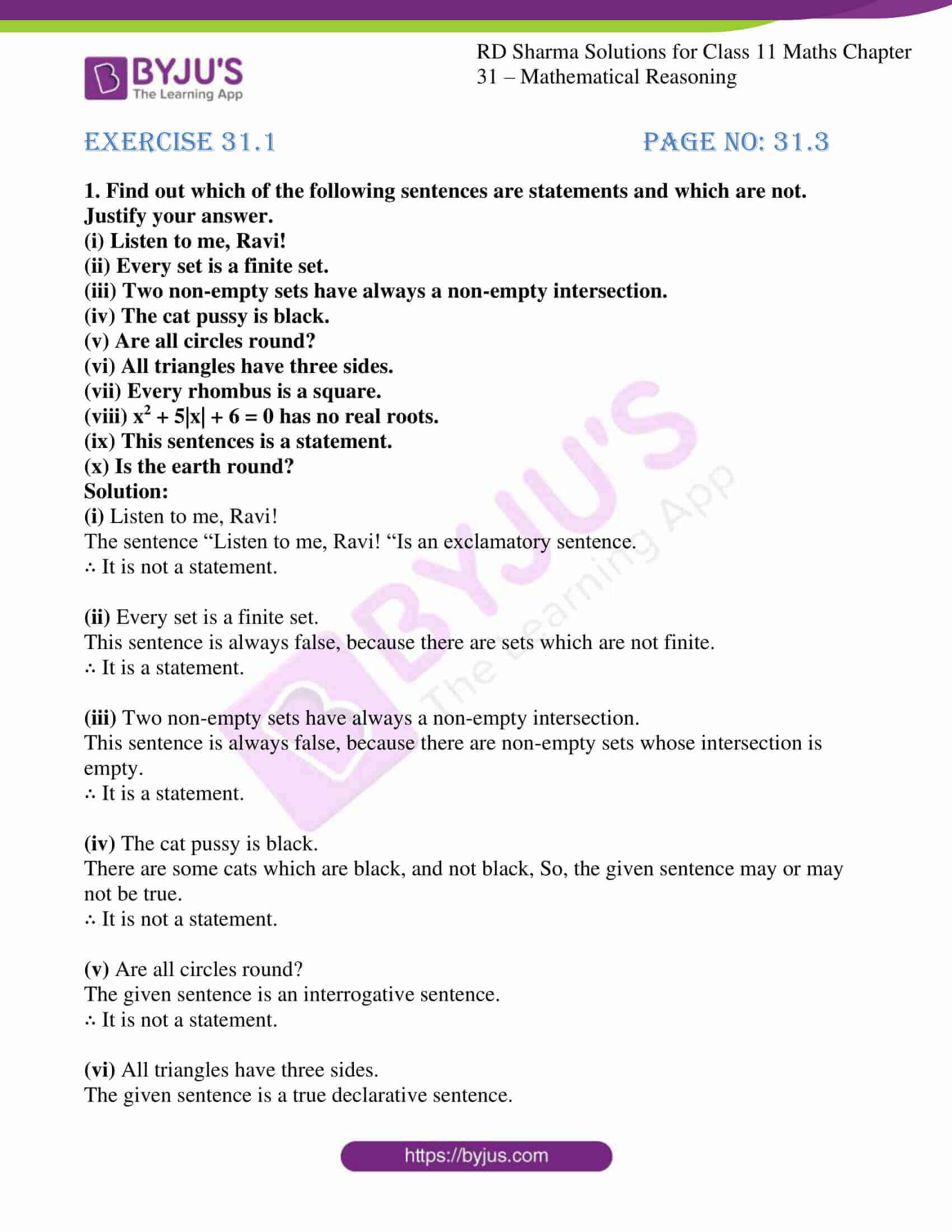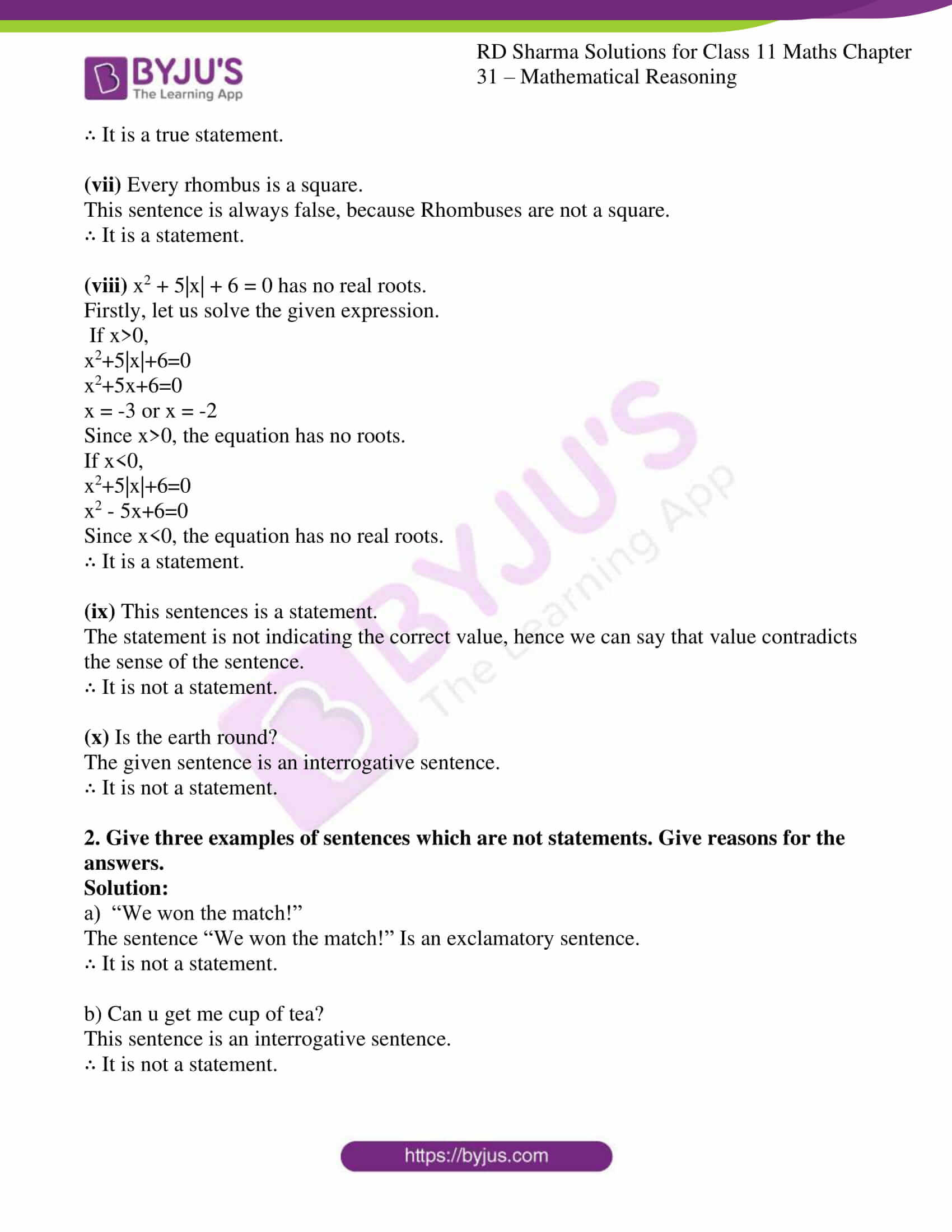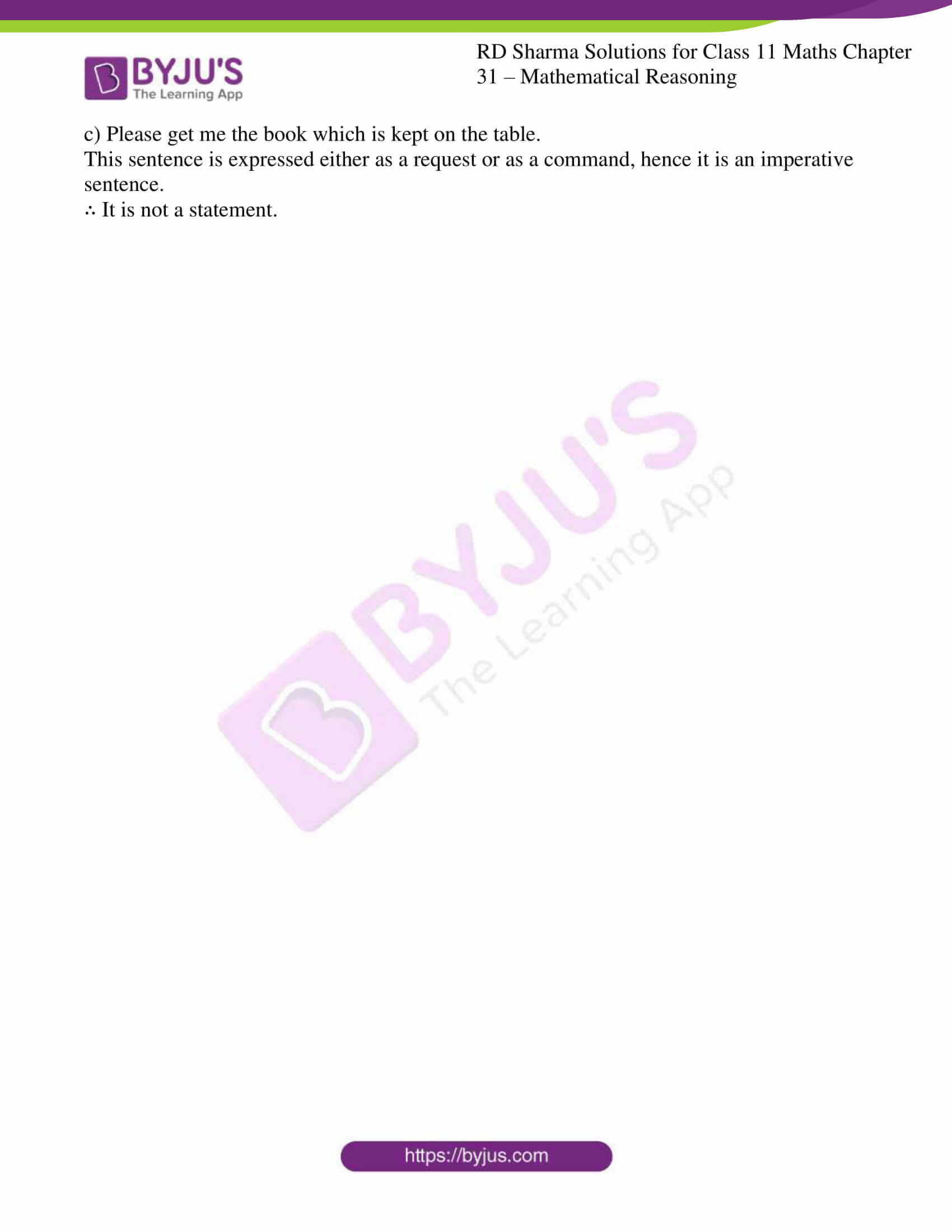# RD Sharma Solutions for Class 11 Chapter 31 - Mathematical Reasoning Exercise 31.1

In this exercise, we shall learn about some basics of mathematical reasoning. The process of reasoning is in the context of mathematics. There are two kinds of reasoning, (i) Inductive reasoning and (ii) Deductive reasoning. The solutions here are made readily available in the pdf format, which helps students ace the exam with good marks. Solving exercise wise problems help students enhance their analytical and problem solving abilities, which are important from the exam point of view. Students can refer to RD Sharma Class 11 Maths Solutions to secure good marks in the board examination. The pdf of RD Sharma Solutions are provided in the links given below, which can be downloaded easily and can be used for future reference as well.

## Download the pdf of RD Sharma Solutions for Class 11 Maths Exercise 31.1 Chapter 31 – Mathematical Reasoning### Access answers to RD Sharma Solutions for Class 11 Maths Exercise 31.1 Chapter 31 – Mathematical Reasoning

#### EXERCISE 31.1 PAGE NO: 31.3

1. Find out which of the following sentences are statements and which are not. Justify your answer.
(i) Listen to me, Ravi!

(ii) Every set is a finite set.

(iii) Two non-empty sets have always a non-empty intersection.

(iv) The cat pussy is black.

(v) Are all circles round?

(vi) All triangles have three sides.

(vii) Every rhombus is a square.

(viii) x2 + 5|x| + 6 = 0 has no real roots.

(ix) This sentences is a statement.

(x) Is the earth round?

Solution:

(i) Listen to me, Ravi!

The sentence “Listen to me, Ravi! “Is an exclamatory sentence.

∴ It is not a statement.

(ii) Every set is a finite set.

This sentence is always false, because there are sets which are not finite.

∴ It is a statement.

(iii) Two non-empty sets have always a non-empty intersection.

This sentence is always false, because there are non-empty sets whose intersection is empty.

∴ It is a statement.

(iv) The cat pussy is black.

There are some cats which are black, and not black, So, the given sentence may or may not be true.

∴ It is not a statement.

(v) Are all circles round?

The given sentence is an interrogative sentence.

∴ It is not a statement.

(vi) All triangles have three sides.

The given sentence is a true declarative sentence.

∴ It is a true statement.

(vii) Every rhombus is a square.

This sentence is always false, because Rhombuses are not a square.

∴ It is a statement.

(viii) x2 + 5|x| + 6 = 0 has no real roots.

Firstly, let us solve the given expression.

If x>0,

x2+5|x|+6=0

x2+5x+6=0

x = -3 or x = -2

Since x>0, the equation has no roots.

If x<0,

x2+5|x|+6=0

x2 – 5x+6=0

Since x<0, the equation has no real roots.

∴ It is a statement.

(ix) This sentences is a statement.

The statement is not indicating the correct value, hence we can say that value contradicts the sense of the sentence.

∴ It is not a statement.

(x) Is the earth round?

The given sentence is an interrogative sentence.

∴ It is not a statement.

2. Give three examples of sentences which are not statements. Give reasons for the answers.

Solution:

a) “We won the match!”

The sentence “We won the match!” Is an exclamatory sentence.

∴ It is not a statement.

b) Can u get me cup of tea?

This sentence is an interrogative sentence.

∴ It is not a statement.

c) Please get me the book which is kept on the table.

This sentence is expressed either as a request or as a command, hence it is an imperative sentence.

∴ It is not a statement.